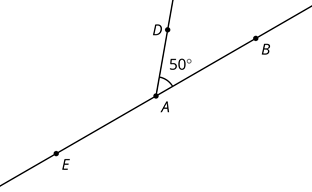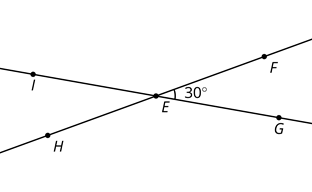# Week 6 Day 1 Opener 19.1What is the measure of angle EAD? What is the measure of angle EAB?What is the measure of angle HEI? What is the measure of angle IEF? What is the measure of angle HEG?